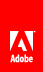# calculate event

#### Description

Initiates in the following situations:

• When your form design and data merge into your finished form.

• When a change occurs to any value that the calculation is dependent on, such as the value of a particular field, unless the form filler has manually overridden the calculated value. As a result, the object will display the return value of the event. The properties for manually overridden fields are located in the Value tab of the Object palette.

• When a field loses focus; for example, when a form filler clicks or uses the Tab key to exit a field.

When using the calculate event to perform calculations or scripts, consider the following potential issues:

• Calculations and scripts on the calculate event must not make any changes to the structure of the form, except for the form field and data values.

• Content inserted by the calculate event must conform to the associated validations for the object; otherwise, validation errors will occur.

• Calculations and scripts must not include an infinite loop because it causes the form to update the value continuously. For example, a script that increments the value of a field as part of a looping expression, such as a while or for loop, could create an infinite loop.

• The last expression evaluated in the calculate event is used to populate the value of the current form object. For example, if the script on the calculate event first sets the value of the current field to 500 and then sets the value of another field to 1000, both fields will display the value 1000 at run time. As a result, you need to limit the scripting that you add to the calculate event to those that deal specifically with setting the value of the current field.

Processing event

#### Support

Client application

Availability

yes

HTML browser

yes

XFA 2.1

#### Example

Use the calculate event for updating numeric values in fields because this event initiates immediately after most other events. For example, on a purchase order form, you can use the calculate event for a field to determine the percentage of sales tax due based on the cost of the order. The calculation will initiate every time a change is made to the values in the form fields, ensuring that the value displayed for the sales tax is always correct.

However, because the calculate event can initiate many times, you must ensure that the calculation or script you add to the event will not cause data values to increment unnecessarily. For example, if your sales tax calculation adds the value of the sales tax to the total cost each time the calculate event initiates, the resulting total cost value on your form may be too large.

For a detailed example of using the calculate event, see Calculating the field sums .// Ethnio survey code removed